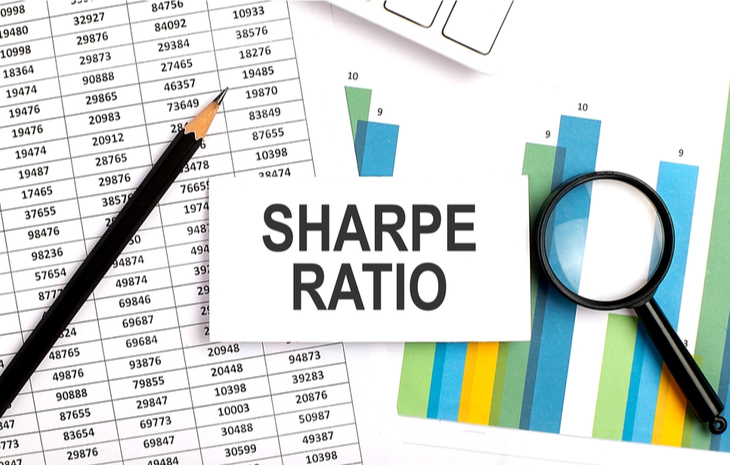Every investment carries with it some level of risk and reward. Unfortunately, these are unknown variables. They change over time and in the face of market factors, and there’s no way of knowing exactly what they’ll be until you realize them. The best investors can do to understand risk and reward is to look at past data to better-identify potential future performance. This is the purpose of the Sharpe Ratio.

The Sharpe Ratio is a metric that measures the risk to reward ratio of a fund or portfolio. Using this ratio, investors can take the past performance of a portfolio and compare it to other investments of the same nature. For instance, if you’re looking at three similar energy ETFs, you can use this ratio to see how they’ve performed relative to each other over the past decade.

The Sharpe Ratio is surprisingly simple to calculate and extremely insightful once you do. Here’s a closer look at this metric, how to calculate it and how investors can apply it to asset valuation.## Understanding the Sharpe Ratio

Conceived by Nobel laureate William F. Sharpe, this metric is a relatively simple metric to understand.

First, it measures the past performance of a fund against the risk-free rate of return. That is to say, how well did the fund perform relative to an investment with virtually no risk whatsoever? Typically, this means stacking up the fund’s returns against a benchmark like U.S. Treasuries. The goal is to understand returns in the context of implied risk.

Speaking of implied risk, the Sharpe Ratio takes the adjusted rate of return for a portfolio and divides it by the standard deviation. Or, in simpler terms, volatility. The ratio itself effectively boils down to a measure of the portfolio’s true performance against risk.

The Sharpe Ratio is risk vs. reward, quantified. This ratio is one of the most popular metrics for calculating risk-adjusted return, especially for followers of the Modern Portfolio Theory. The higher the ratio, the better the performance of the fund or portfolio. The lower the figure, the lower its performance in the face of risk.

## Formula and Calculation

As mentioned, the Sharpe Ratio is fairly easy to calculate. In fact, most managed funds provide this ratio as a convenience to potential investors. If you do want to calculate it yourself, the formula looks like this:

Sharpe Ratio = Rp – Rf / σp

In the above formula:

• Rp = The return of the portfolio
• Rf = The risk-free rate of return
• σp = Standard deviation (risk)

It’s important to establish the standard deviation of the portfolio before calculating the Sharpe Ratio, and to compare it to a current benchmark for risk-free return. Example:

Jim wants to determine the performance of his portfolio over the last 10 years using the Sharpe Ratio. He selects a 4% T-Note as the rate of risk-free return. His portfolio averaged 14% over that time period and has a standard deviation of 20%. Based on these figures, Jim would calculate the Sharpe Ratio as 14% – 4% / 20%, which gives him a ratio of 0.5.

Typically, it’s best to calculate this ratio over an established period of time, such as five or 10 years. Keep in mind, however: the longer the time horizon, the more ineffectual this ratio is as a measure of total fund performance in the face of volatility.

## An Evaluation Metric

Once you have the Sharpe Ratio, what do you do with it? It’s best-used as a comparative metric, usually for mutual funds or ETFs. Remember, the higher the ratio, the better the fund’s performance. Taking a handful of similar funds and comparing the Sharpe Ratio is a great way to understand their risk-adjusted performance at a glance.

For instance, Funds A, B, C and D may all present similarly, with slightly different asset allocations. To understand how that asset allocation affects the risk-reward profile of the fund, investors can look at the Sharpe Ratio. If the ratios are 1.35, 1.43, 1.49 and 1.37, we can assume that Fund C offers the most favorable performance in the face of volatility.

The Sharpe Ratio can tell investors where they’re going to get the best bang for their buck when it comes to volatility. Considering that it’s impossible to predict future performance, this is a valuable metric when it comes to assessing the unknown and how a fund might perform. The ratio tells you if excess returns are the result of good investment decision-making or an appetite for risk.

## Drawbacks of the Sharpe Ratio

The one real drawback of this ratio is that it assumes normal distribution within a portfolio. The denominator (standard deviation) doesn’t account for allocation and thus, is skewed by portfolio weight. Keep this in mind and beware of outliers when looking at a true measure of standard deviation. It can help to plot a bell curve to identify outliers.

The Sharpe Ratio is also easy to manipulate by expanding the window for assessing returns. Time smooths out volatility, which can raise the ratio when looking at fund performance over periods longer than a decade. For instance, the ratio for a five-year period might be 0.85, while that same portfolio may register a ratio of 1.75 over a 20-year horizon.

## What’s a Good Sharpe Ratio?

The Sharpe Ratio is best-used as a comparison metric, which means a good ratio is one that’s highest relative to its peers. That said, anything above 1 is often positive, indicating reward that far exceeds risk. Be sure to always compare like-kind investments and remember that, like all metrics, this one is best-applied in context, alongside broader fundamental analysis or performance.